# Determining the Equation of a Line

In this section, you will learn to:

1. Find an equation of a line if a point and the slope are given.
2. Find an equation of a line if two points are given.

So far, we were given an equation of a line and were asked to give information about it. For example, we were asked to find points on it, find its slope, and find intercepts. Now we are going to reverse the process. That is, we will be given either two points, or a point and the slope of a line, and we will be asked to find its equation.

An equation of a line can be written in two forms, the slope-intercept form or the standard form.

The Slope-Intercept Form of a Line:     y = mx + b

A line is completely determined by two points, or a point and slope. So it makes sense to ask to find the equation of a line if one of these two situations is given.

Example 2.3.1

Find an equation of a line whose slope is 5, and y-intercept is 3.
Solution
In the last section we learned that the equation of a line whose slope = m and y-intercept = b is y = mx + b.
Since m = 5, and b = 3, the equation is y = 5x + 3.

Example 2.3.2

Find the equation of the line that passes through the point (2, 7) and has slope 3.
Solution
Since m = 3, the partial equation is y = 3x + b.
Now b can be determined by substituting the point (2, 7) in the equation y = 3x + b.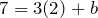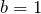Therefore, the equation is y = 3x + 1.

Example 2.3.3

Find an equation of the line that passes through the points (-1, 2), and (1, 8).
Solution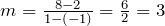So the partial equation is y = 3x + b
Now we can use either of the two points (-1, 2) or (1, 8), to determine b. Substituting (-1, 2) gives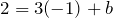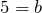So the equation is y = 3x + 5.

Example 2.3.4

Find an equation of the line that has x-intercept 3, and y-intercept 4.
Solution
x-intercept = 3, and y-intercept = 4 correspond to the points (3, 0), and (0, 4), respectively.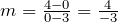So the partial equation for the line is y = – 4/3x + b
Substituting (0, 4) gives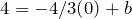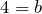Therefore, the equation is y = – 4/3x + 4.

The Standard form of a Line: Ax + By = C
Another useful form of the equation of a line is the Standard form.
Let L be a line with slope m, and containing a point (x1, y1). If (x, y) is any other point on the line L, then by the definition of a slope, we get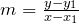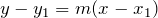The last result is referred to as the point-slope form or point-slope formula. If we simplify this formula, we get the equation of the line in the standard form, Ax + By = C.

Example 2.3.5

Using the point-slope formula, find the standard form of an equation of the line that passes through the point (2, 3) and has slope – 3/5.
Solution
Substituting the point (2, 3) and m = – 3/5 in the point-slope formula, we get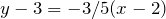Multiplying both sides by 5 gives us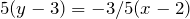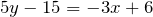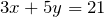Example 2.3.6

Find the standard form of the line that passes through the points (1, -2), and (4, 0).

Solution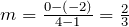The point-slope form is: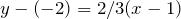Multiplying both sides by 3 gives us: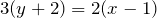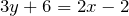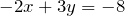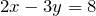We should always be able to convert from one form of an equation to another. That is, if we are given a line in the slope-intercept form, we should be able to express it in the standard form, and vice versa.

Example 2.3.7

Write the equation y = – 2/3x +3 in the standard form.

Solution

Multiplying both sides of the equation by 3, we get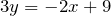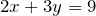Example 2.3.8

Write the equation 3x − 4y = 10 in the slope-intercept form.
Solution
Solving for y, we get: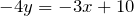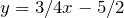Finally, we learn a very quick and easy way to write an equation of a line in the standard form. But first we must learn to find the slope of a line in the standard form by inspection.

By solving for y, it can easily be shown that the slope of the line Ax + By = C is −A/B. The reader should verify.

Example 2.3.9

Find the slope of the following lines, by inspection.
a. 3x − 5y = 10
b. 2x + 7y = 20
c. 4x − 3y = 8
Solution
a. A = 3, B = −5, therefore,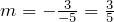b. A = 2, B = 7, therefore,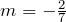c.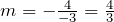Now that we know how to find the slope of a line in the standard form by inspection, our job in finding the equation of a line is going to be very easy.

Example 2.3.10

Find an equation of the line that passes through (2, 3) and has slope −4/5.
Solution
Since the slope of the line is −4/5, we know that the left side of the equation is 4x +5y, and the partial equation is going to be: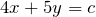Of course, c can easily be found by substituting for x and y.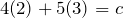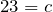The desired equation is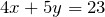.
If you use this method often enough, you can do these problems very quickly.

# Practice questions

1. Write an equation of the line satisfying the following conditions. Write the equation in the form y = mx + b.

a. Passes through (3, 5) and (2, -1).

b. Passes through (5, -2) and m =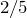.

c. Passes through (2, -5) and its x-intercept is 4.

d. Passes through (-3, -4), and (-5, 2).

e. Is a horizontal line passing through (2, -1).

f. Has an x-intercept = 3 and y-intercept = 4.

2. Write an equation of the line that satisfies the following conditions. Write the equation in the form Ax + By = C.

a. Passes through (-4, -2) and m =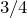b. Passes through (2, -3) and (5, 1).